Search by Topic

Resources tagged with Visualising similar to Ben's Game:

Filter by: Content type:
Age range:
Challenge level:

There are 176 results

Broad Topics > Using, Applying and Reasoning about Mathematics > VisualisingHello Again

Age 11 to 14 Challenge Level:

Anne completes a circuit around a circular track in 40 seconds. Brenda runs in the opposite direction and meets Anne every 15 seconds. How long does it take Brenda to run around the track?Cuboids

Age 11 to 14 Challenge Level:

Find a cuboid (with edges of integer values) that has a surface area of exactly 100 square units. Is there more than one? Can you find them all?Tetrahedra Tester

Age 11 to 14 Challenge Level:

An irregular tetrahedron is composed of four different triangles. Can such a tetrahedron be constructed where the side lengths are 4, 5, 6, 7, 8 and 9 units of length?Diminishing Returns

Age 11 to 14 Challenge Level:

How much of the square is coloured blue? How will the pattern continue?Age 14 to 16 Challenge Level:

Four rods are hinged at their ends to form a convex quadrilateral. Investigate the different shapes that the quadrilateral can take. Be patient this problem may be slow to load.3D Treasure Hunt

Age 14 to 18 Challenge Level:

Some treasure has been hidden in a three-dimensional grid! Can you work out a strategy to find it as efficiently as possible?Jam

Age 14 to 16 Challenge Level:

To avoid losing think of another very well known game where the patterns of play are similar.Fermat's Poser

Age 14 to 16 Challenge Level:

Find the point whose sum of distances from the vertices (corners) of a given triangle is a minimum.Age 14 to 16 Challenge Level:

In this problem we are faced with an apparently easy area problem, but it has gone horribly wrong! What happened?Vanishing Point

Age 14 to 18 Challenge Level:

How can visual patterns be used to prove sums of series?Something in Common

Age 14 to 16 Challenge Level:

A square of area 3 square units cannot be drawn on a 2D grid so that each of its vertices have integer coordinates, but can it be drawn on a 3D grid? Investigate squares that can be drawn.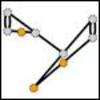Sprouts

Age 7 to 18 Challenge Level:

A game for 2 people. Take turns joining two dots, until your opponent is unable to move.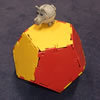Platonic Planet

Age 14 to 16 Challenge Level:

Glarsynost lives on a planet whose shape is that of a perfect regular dodecahedron. Can you describe the shortest journey she can make to ensure that she will see every part of the planet?Tied Up

Age 14 to 16 Short Challenge Level:

How much of the field can the animals graze?Building Tetrahedra

Age 14 to 16 Challenge Level:

Can you make a tetrahedron whose faces all have the same perimeter?Just Rolling Round

Age 14 to 16 Challenge Level:

P is a point on the circumference of a circle radius r which rolls, without slipping, inside a circle of radius 2r. What is the locus of P?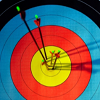Spotting the Loophole

Age 14 to 16 Challenge Level:

A visualisation problem in which you search for vectors which sum to zero from a jumble of arrows. Will your eyes be quicker than algebra?Instant Insanity

Age 11 to 18 Challenge Level:

Given the nets of 4 cubes with the faces coloured in 4 colours, build a tower so that on each vertical wall no colour is repeated, that is all 4 colours appear.Cubic Net

Age 14 to 18 Challenge Level:

This is an interactive net of a Rubik's cube. Twists of the 3D cube become mixes of the squares on the 2D net. Have a play and see how many scrambles you can undo!Ding Dong Bell

Age 11 to 18

The reader is invited to investigate changes (or permutations) in the ringing of church bells, illustrated by braid diagrams showing the order in which the bells are rung.Coloured Edges

Age 11 to 14 Challenge Level:

The whole set of tiles is used to make a square. This has a green and blue border. There are no green or blue tiles anywhere in the square except on this border. How many tiles are there in the set?Cubes Within Cubes

Age 7 to 14 Challenge Level:

We start with one yellow cube and build around it to make a 3x3x3 cube with red cubes. Then we build around that red cube with blue cubes and so on. How many cubes of each colour have we used?Square It

Age 11 to 16 Challenge Level:

Players take it in turns to choose a dot on the grid. The winner is the first to have four dots that can be joined to form a square.Sliding Puzzle

Age 11 to 16 Challenge Level:

The aim of the game is to slide the green square from the top right hand corner to the bottom left hand corner in the least number of moves.Khun Phaen Escapes to Freedom

Age 11 to 14 Challenge Level:

Slide the pieces to move Khun Phaen past all the guards into the position on the right from which he can escape to freedom.Hypotenuse Lattice Points

Age 14 to 16 Challenge Level:

The triangle OMN has vertices on the axes with whole number co-ordinates. How many points with whole number coordinates are there on the hypotenuse MN?Changing Places

Age 14 to 16 Challenge Level:

Place a red counter in the top left corner of a 4x4 array, which is covered by 14 other smaller counters, leaving a gap in the bottom right hand corner (HOME). What is the smallest number of moves. . . .Sliced

Age 14 to 16 Challenge Level:

An irregular tetrahedron has two opposite sides the same length a and the line joining their midpoints is perpendicular to these two edges and is of length b. What is the volume of the tetrahedron?Icosian Game

Age 11 to 14 Challenge Level:

This problem is about investigating whether it is possible to start at one vertex of a platonic solid and visit every other vertex once only returning to the vertex you started at.Inside Out

Age 14 to 16 Challenge Level:

There are 27 small cubes in a 3 x 3 x 3 cube, 54 faces being visible at any one time. Is it possible to reorganise these cubes so that by dipping the large cube into a pot of paint three times you. . . .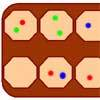Wari

Age 14 to 16 Challenge Level:

This is a simple version of an ancient game played all over the world. It is also called Mancala. What tactics will increase your chances of winning?Jam

Age 14 to 16 Challenge Level:

A game for 2 playersClocked

Age 11 to 14 Challenge Level:

Is it possible to rearrange the numbers 1,2......12 around a clock face in such a way that every two numbers in adjacent positions differ by any of 3, 4 or 5 hours?Picturing Triangular Numbers

Age 11 to 14 Challenge Level:

Triangular numbers can be represented by a triangular array of squares. What do you notice about the sum of identical triangle numbers?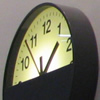Clocking Off

Age 7 to 16 Challenge Level:

I found these clocks in the Arts Centre at the University of Warwick intriguing - do they really need four clocks and what times would be ambiguous with only two or three of them?LOGO Challenge - Triangles-squares-stars

Age 11 to 16 Challenge Level:

Can you recreate these designs? What are the basic units? What movement is required between each unit? Some elegant use of procedures will help - variables not essential.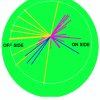Charting Success

Age 11 to 16 Challenge Level:

Can you make sense of the charts and diagrams that are created and used by sports competitors, trainers and statisticians?Coke Machine

Age 14 to 16 Challenge Level:

The coke machine in college takes 50 pence pieces. It also takes a certain foreign coin of traditional design...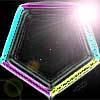Penta Colour

Age 14 to 16 Challenge Level:

In how many different ways can I colour the five edges of a pentagon red, blue and green so that no two adjacent edges are the same colour?Squares in Rectangles

Age 11 to 14 Challenge Level:

A 2 by 3 rectangle contains 8 squares and a 3 by 4 rectangle contains 20 squares. What size rectangle(s) contain(s) exactly 100 squares? Can you find them all?Double Trouble

Age 14 to 16 Challenge Level:

Simple additions can lead to intriguing results...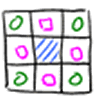Thinking Through, and By, Visualising

Age 7 to 16

This article is based on some of the ideas that emerged during the production of a book which takes visualising as its focus. We began to identify problems which helped us to take a structured view. . . .Contact

Age 14 to 16 Challenge Level:

A circular plate rolls in contact with the sides of a rectangular tray. How much of its circumference comes into contact with the sides of the tray when it rolls around one circuit?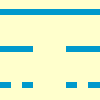The Cantor Set

Age 11 to 14 Challenge Level:

Take a line segment of length 1. Remove the middle third. Remove the middle thirds of what you have left. Repeat infinitely many times, and you have the Cantor Set. Can you picture it?Triangles in the Middle

Age 11 to 18 Challenge Level:

This task depends on groups working collaboratively, discussing and reasoning to agree a final product.When the Angles of a Triangle Don't Add up to 180 Degrees

Age 14 to 18

This article outlines the underlying axioms of spherical geometry giving a simple proof that the sum of the angles of a triangle on the surface of a unit sphere is equal to pi plus the area of the. . . .Cogs

Age 11 to 14 Challenge Level:

A and B are two interlocking cogwheels having p teeth and q teeth respectively. One tooth on B is painted red. Find the values of p and q for which the red tooth on B contacts every gap on the. . . .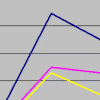Charting More Success

Age 11 to 16 Challenge Level:

Can you make sense of the charts and diagrams that are created and used by sports competitors, trainers and statisticians?Prime Magic

Age 7 to 16 Challenge Level:

Place the numbers 1, 2, 3,..., 9 one on each square of a 3 by 3 grid so that all the rows and columns add up to a prime number. How many different solutions can you find?Just Opposite

Age 14 to 16 Challenge Level:

A and C are the opposite vertices of a square ABCD, and have coordinates (a,b) and (c,d), respectively. What are the coordinates of the vertices B and D? What is the area of the square?